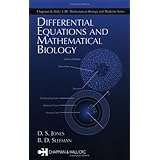Differential Equations and Mathematical Biology The conjoining of mathematics and biology has brought about significant advances in both areas, with mathematics providing a tool for modelling and understanding biological phenomena and biology stimulating developments in the theory of nonlinear differential equations. The continued application of mathematics to biology holds great promise and in fact may be the applied mathematics of the 21st century.Differential Equations and Mathematical Biology provides a detailed treatment of both ordinary and partial differential equations, techniques for their solution, and their use in a variety of biological applications. The presentation includes the fundamental techniques of nonlinear differential equations, bifurcation theory, and the impact of chaos on discrete time biological modelling. The authors provide generous coverage of numerical techniques and address a range of important applications, including heart physiology, nerve pulse transmission, chemical reactions, tumour growth, and epidemics.This book is the ideal vehicle for introducing the challenges of biology to mathematicians and likewise delivering key mathematical tools to biologists. Carefully designed for such multiple purposes, it serves equally well as a professional reference and as a text for coursework in differential equations, in biological modelling, or in differential equation models of biology for life science students.